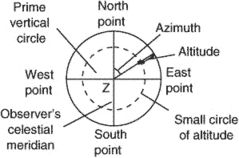# altitude

(redirected from altatude)
Also found in: Dictionary.

## altitude,

vertical distance of an object above some datum plane, such as mean sea levelsea level,
the level of the sea, which serves as the datum used for measurement of land elevations and ocean depths. Theoretically, one would expect sea level to be a fixed and permanent horizontal surface on the face of the earth, and as a starting approximation, this is true.
or a reference point on the earth's surface. It is usually measured by the reduction in atmospheric pressure with height, as shown on a barometerbarometer
, instrument for measuring atmospheric pressure. It was invented in 1643 by the Italian scientist Evangelista Torricelli, who used a column of water in a tube 34 ft (10.4 m) long.
or altimeteraltimeter
, device for measuring altitude. The most common type is an aneroid barometer calibrated to show the drop in atmospheric pressure in terms of linear elevation as an airplane, balloon, or mountain climber rises.
. In surveying and astronomy, it is the vertical angle of an observed point, such as a star or planet, above the horizon plane. The altitude of a feature of the earth's surface is usually called its elevationelevation,
vertical distance from a datum plane, usually mean sea level to a point above the earth. Often used synonymously with altitude, elevation is the height on the earth's surface and altitude, the height in space above the surface.
. Recent spacecraft instrumentation has also measured vertical distances on the earth and other planets, determining the height of planetary features by means of radar and optical imaging.

In astronomy, altitude is the angular distance of a heavenly body above the astronomical horizon as determined by the angle which a line drawn from the eye of the observer to the heavenly body makes with the plane of the horizon. The reading of the apparent altitude, as determined by a telescope attached to a graduated circle, must be corrected for refraction by the atmosphere and for certain other effects to ascertain the true altitude. The altitude of the north celestial pole, which is approximately that of the star PolarisPolaris
or North Star,
star nearest the north celestial pole (see equatorial coordinate system). It is in the constellation Ursa Minor (see Ursa Major and Ursa Minor; Bayer designation Alpha Ursae Minoris) and marks the end of the handle of the Little Dipper.
, is equal to the observer's latitude.

## altitude

The angular distance of a point or celestial object above or below the horizon, or of an object, such as an artificial satellite, above mean sea level. Altitude and azimuth are coordinates in the horizontal coordinate system.
Collins Dictionary of Astronomy © Market House Books Ltd, 2006

## Altitude

(religion, spiritualism, and occult)

In astrology, altitude refers to the angular distance (i.e., measured in degrees of an arc) that a point, planet, or other heavenly body is situated above or below the horizon. Above the horizon, altitude is measured up to a maximum angular distance of 90° (directly overhead); below the horizon, down to a maximum of-90° (directly underneath).

The following article is from The Great Soviet Encyclopedia (1979). It might be outdated or ideologically biased.

## Altitude

the angular displacement of an observed object, such as a flight vehicle or a celestial body, above the celestial horizon. In astronomy, altitude is used together with azimuth to determine the direction to an object in the horizon system of coordinates.

## altitude

[′al·tə‚tüd]
Abbreviated alt.
(engineering)
Height, measured as distance along the extended earth's radius above a given datum, such as average sea level.
Angular displacement above the horizon measured by an altitude curve.
(mathematics)
The perpendicular distance from the base to the top (a vertex or parallel line) of a geometric figure such as a triangle or parallelogram.
McGraw-Hill Dictionary of Scientific & Technical Terms, 6E, Copyright © 2003 by The McGraw-Hill Companies, Inc.

## altitudeAltitude (celestial).
.
i. The vertical distance of a level, a point, or an object considered as a point, measured from mean sea level (ICAO). In the case of aircraft in flight, it is normally expressed in flight levels or hundreds of feet. For example, an aircraft flying at 25,000 ft AMSL (above mean sea level) would be said to be flying at flight level 250. A barometric altimeter shows pressure altitude. When it is corrected for instrument errors and necessary compensation is made for variation from standard atmospheric conditions, it is called a true altitude. Absolute altitude is measured by a radio, radar, or laser altimeter. Absolute altitude is the true distance from the surface of the earth at that point. In some definitions, heights, which are the vertical distance of an object from an object, point, or level above ground or other reference plane, are also called altitude, such as in AGL (above ground level) altitude, MSL (mean seal level) altitude, and indicated altitude (that indicated by an altimeter).
ii. The angular displacement above the horizon; the arc of a vertical circle between the horizon and a point on the celestial sphere measured upward from the horizon from 0° to 90°. Angular displacement below the horizon is called negative altitude, or dip. See also computed altitude and density altitude.